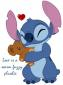# equation of the circle

2 posts / 0 new
Etteneaj Orachabequation of the circle

find the equation of the circle tangent to 3x+y+14=0 and x+3y+10=0 w/ radius square root of 10.
please someone Help me in this equations I really do need Badly some help.

fitzmerl duronTo get the equation of the circle, minding those conditions, we need to make a figure...Recall that the distance from the line $Ax_1+By_1+C=0$ to a point $P(x,y)$ (or from a point $P(x,y)$ to a line $Ax+By+C=0$) is:

$$d = \frac{Ax_1+By_1+C}{\pm \sqrt{A^2 + B^2}}$$

and the sign of the radical $\sqrt{A^2 + B^2}$ must be opposite that of $C$.

So...

We let $r_1$ and $r_2$ be the radius of the circle. It is the distance from the lines $\color{red}{3x+y + 14 =0}$ and $\color{blue}{x+3y+10=0}$, respectively, to a center of this future circle.

Then...

$$r_1 = -r_2$$

For $r_1$:

$$d = \frac{Ax_1+By_1+C}{\pm \sqrt{A^2 + B^2}}$$ $$r_1 = \frac{Ax_0+By_0+C}{\pm \sqrt{A^2 + B^2}}$$ $$\sqrt{10} = \frac{3x_0+1y_0+14}{-\sqrt{(3)^2 + (1)^2}}$$ $$\sqrt{10} = \frac{3x_0+1y_0+14}{-\sqrt{10}}$$ $$-10 = 3x_0 + 1y_0 + 14$$ $$3x_0 + y_0 = -24$$

For $r_2$:

$$d = \frac{Ax_1+By_1+C}{\pm \sqrt{A^2 + B^2}}$$ $$-r_2 = \frac{Ax_0+By_0+C}{\pm \sqrt{A^2 + B^2}}$$ $$-\sqrt{10} = \frac{x_0+3y_0+10}{-\sqrt{(1)^2 + (3)^2}}$$ $$-\sqrt{10} = \frac{x_0+3y_0+10}{-\sqrt{10}}$$ $$-10 = x_0 + 3y_0 + 10$$ $$1x_0 + 3y_0 = 0$$

Note: the reason why I equate $r_1$ to the negative of $r_2$ is because I was looking for the equation of this circle that is tangent to the lines $\color{red}{3x+y +14 =0}$ and $\color{blue}{x+3y+10=0}$ and the center of this circle will be situated along the equation of the acute angle bisector between the lines $\color{red}{3x+y + 14 =0}$ and $\color{blue}{x+3y+10=0}$. If you are wondering what is the acute angle bisector of the given two lines, it is:

$$d = -d'$$ $$\frac{3x+y+14}{-\sqrt{3^2+1^2}} = -\frac{x+3y+10}{-\sqrt{1^2 + 3^2}}$$ $$-(3x+y+14) = x+3y+10$$ $$-3x-y-14 = x+3y+10$$ $$-4x-4y-24 =0$$ $$\color{green}{x+y+6=0}$$

Getting the $y$, it is: $\color{green}{y = -x-6}$Getting the unknowns of two equations $3x_0 +y_0 = -24$ and $x_0 + 3y_0 = 0$, the unknowns are $x_0 = -9 \space and \space y_0 = 3$. Those are the coordinates for the center of your circle.

Therefore, the equation of your circle is $$\color{orange}{(x+9)^2 + (y-3)^2 = (\sqrt{10})^2}$$

Solving for its $y$, it becomes $$\color{orange}{y = 3 \pm \sqrt{10 - (x+9)^2}}$$Alternate solutions are encouraged....:-)

• Mathematics inside the configured delimiters is rendered by MathJax. The default math delimiters are $$...$$ and $...$ for displayed mathematics, and $...$ and $...$ for in-line mathematics.